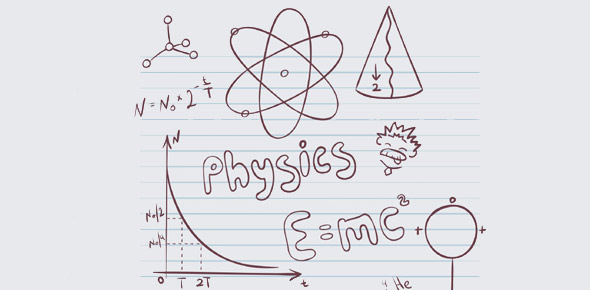# Physics Quiz: Energy And Force

10 Questions | Attempts: 203SettingsLet’s get down to business in this quiz on the scientific study of physics, as we turn our attention to such topics as conduction, repulsion, electricity, force, static energy, and much more. What can you tell us?

• 1.
Two styrofoam packing beads suspended from threads show a slight repulsion away from each other. from this we can infer that:
• A.

• B.

At least one of the beads must be charged

• C.

Both beads must be charged and with the same sign charge.

• D.

Both beads must be charged and with opposite sign charges.

• E.

None of these

• 2.
A neutral, conducting sphere mounted on an insulting stand. A grounded wire is touched to the sphere. A charged rod is brought near the sphere; the wire is removed and then the rod is removed. the sphere is left with an electrical charge that is:
• A.

Neutral

• B.

Opposite that of the charged rod

• C.

The same as that of the charged rod.

• D.

- if the rod was -, but neutral if the rod was +

• E.

+ if the rod was + but neutral if the rod was -

• 3.
Neutral objects such as bits of straw will be:
• A.

Weakly attracted by - charged objects

• B.

Weakly attracted by + charged objects

• C.

Weakly repelled by neutral objects

• D.

Both a and b

• E.

All correct

• 4.
Consider two point charges that are separated by a distance 2r. If this distance between them is increased to 5r, the force between the charges is:
• A.

1/25 as great as it had been

• B.

4/25 as great as it had been

• C.

1/9 as great as it had been

• D.

25 times as great as it had been

• E.

None of these

• 5.
An electric field:
• A.

Surrounds a charge

• B.

Is said to exist in a region where a net force will act upon a stationary charge

• C.

Is where corn and soybeans are grown beneath high voltage transmission lines

• D.

Both a and b

• E.

None of these

• 6.
The electric field is zero:
• A.

Inside any conductor

• B.

Inside any conductor with a static charge

• C.

Inside any material, conductor, or insulator with a static charge.

• D.

Never

• E.

Always

• 7.
If there is a force of 5.0E-12N acting to the left on an electron, the electric field intensity at the location of this electron will be:
• A.

Zero

• B.

8.0E-31 n/c to the left

• C.

3.1E7 n/c to the left

• D.

3.1E7 n/c to the right

• E.

None of these

• 8.
The arrows below could represent the pattern of an electric field around:
• A.

A positive ion at the center

• B.

Any "point" charge at the center.

• C.

An electron or a negative ion at the center

• D.

A dipole, a + and - charge pair, since the electric field goes both left and right, both up and down.

• E.

None of these.

• 9.
Consider a metal statue whose shape is shown below. If there is a static charge upon this object, the electric field intensity will be strongest:
• A.

Near the surface near a

• B.

Near the surface near b

• C.

With a static charge, the electric field intensity will be uniform around a conductor.

• D.

It will be strongest near a if the object is charged - and strongest near b if it charged +

• E.

It will be strongest near b if the object is charged - and strongest near a if it charged +

• 10.
The molecular chains of DNA double helix are held together by bonds between adenine and thymine and the other base pair, cytosine and guanaine. What is the force involved if one of these bonds is modeled as an electron 1.8E-10 m away from a proton?
• A.

It is less than 10 - 10 N

• B.

7.1E -9 N

• C.

4.4 E -6 N

• D.

8.0 N

• E.

It is more than 10^2 N

## Related TopicsBack to top
×

Wait!
Here's an interesting quiz for you.